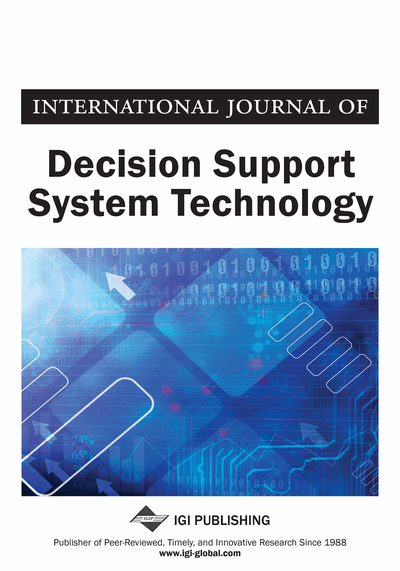# Extended TOPSIS with Correlation Coefficient of Triangular Intuitionistic Fuzzy Sets for Multiple Attribute Group Decision Making

John Robinson P. (Bishop Heber College, India) and Henry AmirtharajE. C. (Bishop Heber College, India)
DOI: 10.4018/jdsst.2011070102

## Abstract

This paper extends the technique for order preference by similarity to ideal solution (TOPSIS) for solving multi-attribute group decision making (MAGDM) problems under triangular intuitionistic fuzzy sets by using its correlation coefficient. In situations where the information or the data is of the form of triangular intuitionistic fuzzy numbers (TIFNs), some arithmetic aggregation operators have to be defined, namely the triangular intuitionistic fuzzy ordered weighted averaging (TIFOWA) operator and the triangular intuitionistic fuzzy hybrid aggregation (TIFHA) operator. An extended TOPSIS model is developed to solve the MAGDM problems using a new type of correlation coefficient defined for TIFNs based on the triangular intuitionistic fuzzy weighted arithmetic averaging (TIFWAA) operator and the TIFHA operator. With an illustration this proposed model of MAGDM with the correlation coefficient of TIFNs is compared with the other existing methods.
Article Preview
Top

## Introduction

Multi-attribute group decision making (MAGDM) problems are of importance in most kinds of fields such as engineering, economics and management. It is obvious that much knowledge in the real world is fuzzy rather than precise. Imprecision comes from a variety of sources such as unquantifiable information (Li & Nan, 2011). In many situations decision makers have imprecise/vague information about alternatives with respect to attributes. One of the methods which describe imprecise cases is the fuzzy set (FS) introduced by Zadeh (1965). It is well known that the conventional decision making analysis using different techniques and tools has been found to be inadequate to handle uncertainty of fuzzy data. To overcome this problem, the concept of fuzzy approach has been used in the evaluation of decision making systems. For a long period of time, efforts have been made in designing various decision making systems suitable for the arising day-to-day problems. Multi attribute group decision making (MAGDM) problems are wide spread in real life decision making situations. A MAGDM problem is to find a desirable solution from a finite number of feasible alternatives assessed on multiple attributes, both quantitative and qualitative. In order to choose a desirable solution, the decision maker often provides his/her preference information which takes the form of numerical values, such as exact values, interval number values and fuzzy numbers. However, under many conditions, numerical values are inadequate or insufficient to model real-life decision problems. Indeed, human judgments including preference information may be stated in intuitionistic fuzzy information, especially in triangular intuitionistic fuzzy information. Hence, MAGDM problems under an intuitionistic fuzzy or a triangular intuitionistic fuzzy environment is an interesting area of study for researchers in the recent days.

In the process of MAGDM problems with triangular intuitionistic fuzzy information, sometimes, the attribute values take the form of Triangular Intuitionistic Fuzzy Number (TIFN). The information about attribute weights may sometimes be known or partially known or sometimes completely unknown. This may be because of the lack of knowledge of the data or the expert’s limited expertise about the problem domain. The MAGDM model requires that the selection be made among the decision alternatives described by their attributes. MAGDM problems are assumed to have a predetermined, limited number of decision alternatives. Solving a MAGDM problem involves sorting and ranking, and can be viewed as alternative methods for combining the information in a problem’s decision matrix together with additional information from the decision maker to determine a final ranking or selection from among the alternatives. Besides the information contained in the decision matrix, all but the simplest MAGDM techniques require additional information from the decision matrix to arrive at a final ranking or selection.

## Complete Article List

Search this Journal:
Reset
Open Access Articles
Volume 12: 4 Issues (2020): 1 Released, 3 Forthcoming
Volume 11: 4 Issues (2019)
Volume 10: 4 Issues (2018)
Volume 9: 4 Issues (2017)
Volume 8: 4 Issues (2016)
Volume 7: 4 Issues (2015)
Volume 6: 4 Issues (2014)
Volume 5: 4 Issues (2013)
Volume 4: 4 Issues (2012)
Volume 3: 4 Issues (2011)
Volume 2: 4 Issues (2010)
Volume 1: 4 Issues (2009)
View Complete Journal Contents Listing